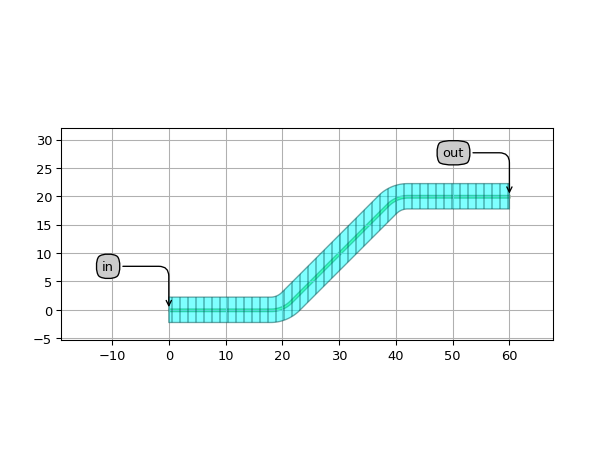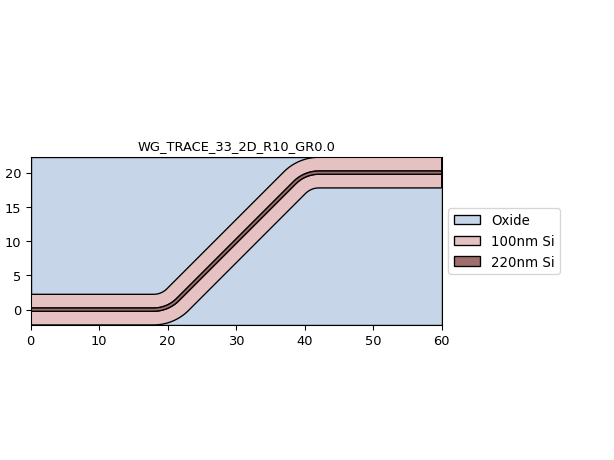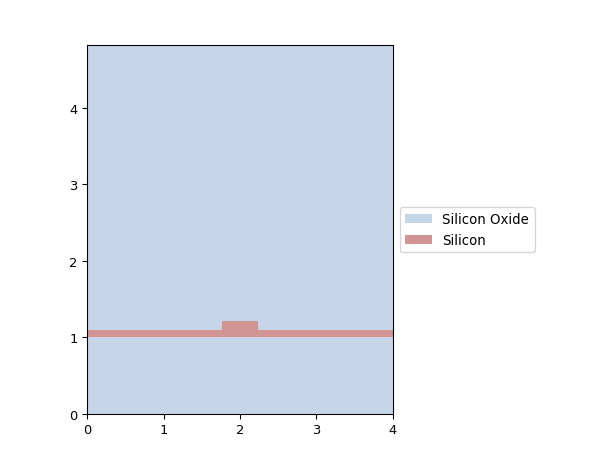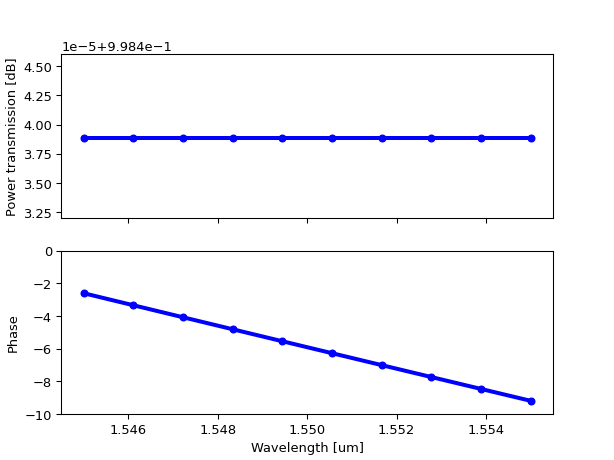# Waveguides¶

## Wire waveguide¶

SiFab contains a silicon ‘wire’ waveguide, which has a rectangular cross-section fully etched through the silicon layer.

• The waveguide template SiWireWaveguideTemplate is parametric in width and wavelength. It contains all the cross-sectional information about the wire waveguide: how to draw the layout, the simulation model parameters like effective and group indices, and so forth.
• Several fixed-width templates are defined: SWG450, SWG800, SWG1000.

### Dispersion diagram¶

We can plot the effective and group indices of the waveguide as a function of width as follows:

# Plot waveguide template dispersion diagram: neff/ng as function of width

import si_fab.all as pdk
import ipkiss3.all as i3
import numpy as np
import pylab as plt

center_env = i3.Environment(wavelength=1.55)
widths = np.linspace(0.4, 1.2, 100)
neffs = []
ngs = []

for w in widths:
tmpl = pdk.SiWireWaveguideTemplate()
tmpl.Layout(core_width=w)
tmpl_cm = tmpl.CircuitModel()
neffs.append(tmpl_cm.get_n_eff(center_env))
ngs.append(tmpl_cm.get_n_g(center_env))

fig, ax1 = plt.subplots()
ax1.plot(widths, neffs, 'bo-', markersize=5, linewidth=3, label='neff')
ax1.set_xlabel('width [um]', fontsize=14)
ax1.set_ylabel('neff', color='blue', fontsize=14)
ax1.tick_params(axis='y', labelcolor='blue', labelsize=14)
ax1.tick_params(axis='x', labelcolor='black', labelsize=14)
ax2 = ax1.twinx()
ax2.plot(widths, ngs, 'ro-', markersize=5, linewidth=3, label='ng')
ax2.set_ylabel('ng', color='red', fontsize=14)
ax2.tick_params(axis='y', labelcolor='red', labelsize=14)
fig.tight_layout()
plt.show()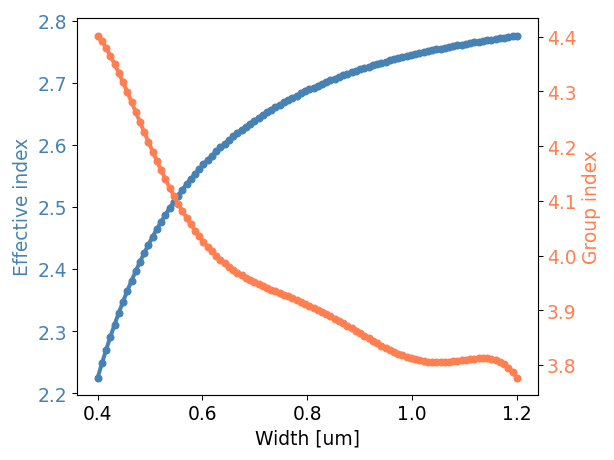### Waveguide layout, virtual fabrication and transmission¶

Based on the waveguide template, we can define a waveguide following a given shape, plot its layout and virtual fabrication (top-down and cross-section). We also plot its power and phase transmission at 1.55 um wavelength:

# Plot layout, cross-section and transmission of a silicon wire waveguide

import si_fab.all as pdk
import ipkiss3.all as i3
import numpy as np
import pylab as plt

center_env = i3.Environment(wavelength=1.55)

# waveguide template: contains all the cross-sectional information.
wg_tmpl = pdk.SiWireWaveguideTemplate()
wg_tmpl.Layout(core_width=0.47)
wg_tmpl_cm = wg_tmpl.CircuitModel()

print("neff @ 1.55: {}".format(wg_tmpl_cm.get_n_eff(center_env)))
print("ng @ 1.55: {}".format(wg_tmpl_cm.get_n_g(center_env)))
print("loss @ 1.55: {}".format(wg_tmpl_cm.get_loss_dB_m(center_env)))

# show an example waveguide layout and virtual fabrication
wg = i3.RoundedWaveguide(trace_template=wg_tmpl)
wg_lo = wg.Layout(shape=[(0.0, 0.0), (20.0, 0.0), (40.0, 20.0), (60.0, 20.0)])
wg_lo.visualize(annotate=True)
wg_lo.visualize_2d(process_flow=i3.TECH.VFABRICATION.PROCESS_FLOW_FEOL)
xs = wg_lo.cross_section(cross_section_path=i3.Shape([(1.0, 2.0), (1.0, -2.0)]))
xs.visualize()
wg_cm = wg.CircuitModel()

# plot the power and phase transmission of this waveguide
wavelengths = np.linspace(1.545, 1.555, 10)
wg_S = wg_cm.get_smatrix(wavelengths)
transmission = wg_S["out", "in"]
plt.figure()
plt.subplot(211)
plt.plot(wavelengths, np.abs(transmission)**2, 'bo-', markersize=5, linewidth=3)
plt.xlabel('wavelength [um]')
plt.ylabel('power transmission')
plt.subplot(212)
plt.plot(wavelengths, np.unwrap(np.angle(transmission)), 'bo-', markersize=5, linewidth=3)
plt.xlabel('wavelength [um]')
plt.ylabel('phase')
plt.show()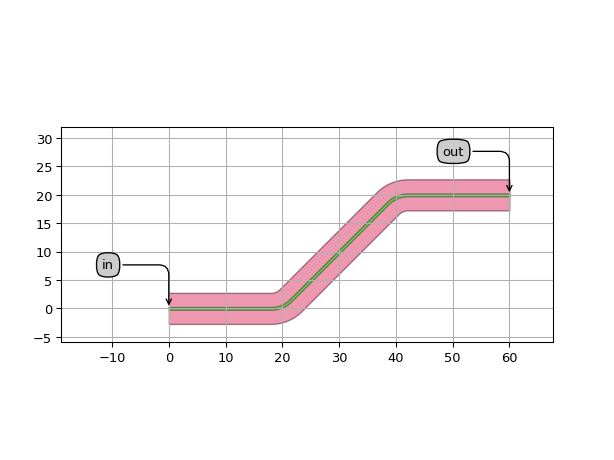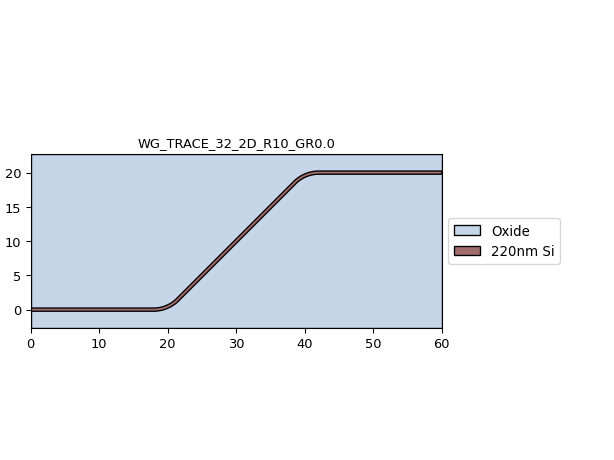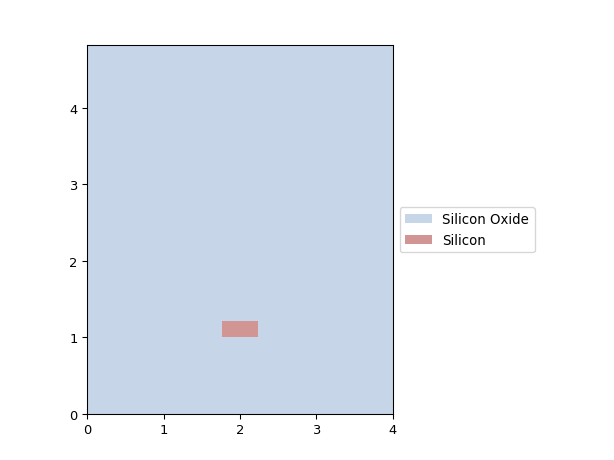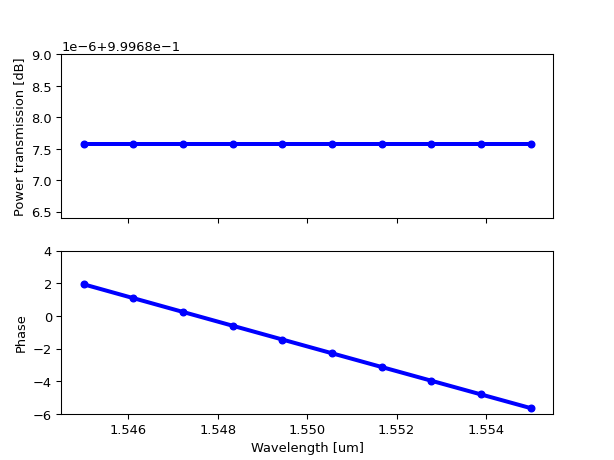## Rib waveguide¶

SiFab contains a silicon ‘rib’ waveguide, which has a rectangular cross-section shallow etched through the silicon layer.

• The waveguide template SiRibWaveguideTemplate is parametric in width and wavelength. It contains all the cross-sectional information about the wire waveguide: how to draw the layout, the simulation model parameters like effective and group indices, and so forth.
• Several fixed-width templates are defined: RWG450, RWG800, RWG1000.

### Dispersion diagram¶

We can plot the effective and group indices of the waveguide as a function of width as follows:

# Plot waveguide template dispersion diagram: neff/ng as function of width

import si_fab.all as pdk
import ipkiss3.all as i3
import numpy as np
import pylab as plt

center_env = i3.Environment(wavelength=1.55)
widths = np.linspace(0.4, 1.2, 100)
neffs = []
ngs = []

for w in widths:
tmpl = pdk.SiRibWaveguideTemplate()
tmpl.Layout(core_width=w)
tmpl_cm = tmpl.CircuitModel()
neffs.append(tmpl_cm.get_n_eff(center_env))
ngs.append(tmpl_cm.get_n_g(center_env))

fig, ax1 = plt.subplots()
ax1.plot(widths, neffs, 'bo-', markersize=5, linewidth=3, label='neff')
ax1.set_xlabel('width [um]', fontsize=14)
ax1.set_ylabel('neff', color='blue', fontsize=14)
ax1.tick_params(axis='y', labelcolor='blue', labelsize=14)
ax1.tick_params(axis='x', labelcolor='black', labelsize=14)
ax2 = ax1.twinx()
ax2.plot(widths, ngs, 'ro-', markersize=5, linewidth=3, label='ng')
ax2.set_ylabel('ng', color='red', fontsize=14)
ax2.tick_params(axis='y', labelcolor='red', labelsize=14)
fig.tight_layout()
plt.show()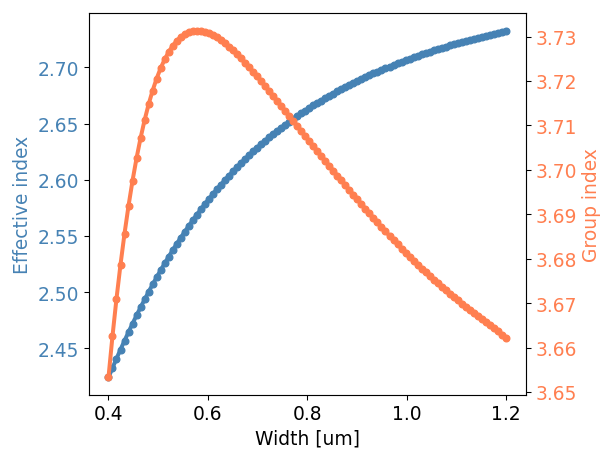### Waveguide layout, virtual fabrication and transmission¶

Based on the waveguide template, we can define a waveguide following a given shape, plot its layout and virtual fabrication (top-down and cross-section). We also plot its power and phase transmission at 1.55 um wavelength:

# Plot layout, cross-section and transmission of a silicon wire waveguide

import si_fab.all as pdk
import ipkiss3.all as i3
import numpy as np
import pylab as plt

center_env = i3.Environment(wavelength=1.55)

# waveguide template: contains all the cross-sectional information.
wg_tmpl = pdk.SiRibWaveguideTemplate()
wg_tmpl.Layout(core_width=0.47)
wg_tmpl_cm = wg_tmpl.CircuitModel()

print("neff @ 1.55: {}".format(wg_tmpl_cm.get_n_eff(center_env)))
print("ng @ 1.55: {}".format(wg_tmpl_cm.get_n_g(center_env)))
print("loss @ 1.55: {}".format(wg_tmpl_cm.get_loss_dB_m(center_env)))

# show an example waveguide layout and virtual fabrication
wg = i3.RoundedWaveguide(trace_template=wg_tmpl)
wg_lo = wg.Layout(shape=[(0.0, 0.0), (20.0, 0.0), (40.0, 20.0), (60.0, 20.0)])
wg_lo.visualize(annotate=True)
wg_lo.visualize_2d(process_flow=i3.TECH.VFABRICATION.PROCESS_FLOW_FEOL)
xs = wg_lo.cross_section(cross_section_path=i3.Shape([(1.0, 2.0), (1.0, -2.0)]))
xs.visualize()
wg_cm = wg.CircuitModel()

# plot the power and phase transmission of this waveguide
wavelengths = np.linspace(1.545, 1.555, 10)
wg_S = wg_cm.get_smatrix(wavelengths)
transmission = wg_S["out", "in"]
plt.figure()
plt.subplot(211)
plt.plot(wavelengths, np.abs(transmission)**2, 'bo-', markersize=5, linewidth=3)
plt.xlabel('wavelength [um]')
plt.ylabel('power transmission')
plt.subplot(212)
plt.plot(wavelengths, np.unwrap(np.angle(transmission)), 'bo-', markersize=5, linewidth=3)
plt.xlabel('wavelength [um]')
plt.ylabel('phase')
plt.show()